# 1st PUC Maths Model Question Paper 3 with Answers

Students can Download 1st PUC Maths Model Question Paper 3 with Answers, Karnataka 1st PUC Maths Model Question Paper with Answers helps you to revise the complete Karnataka State Board Syllabus and score more marks in your examinations.

## Karnataka 1st PUC Maths Model Question Paper 3 with Answers

Time: 3 Hrs 15 Min
Max. Marks: 100

Instructions:

1. The question paper has five parts namely A, B, C, D and E. Answer all the parts.
2. Part-A carries 10 marks. Part-B carries 20 marks. Part-C carries 30 marks. Part-D carries 20 marks, Part-E carries 10 marks.

Part – A

Answer ALL the questions. Each question carries one mark. (10 × 1 = 10)

Question 1.
Write the set {x : x ∈ R and -4< x ≤ 6) as an interval.
{-3, -2, -1,0,1,2,3,4,5,6} ∴ x ∈ (-3,6)]

Question 2.
If (x + 1, y – 2) = (3,1), find the value of x and y.
x + 1 = 3, ∴ x = 2
y – 2 = 1, ∴ y = 3.

Question 3.
Convert $$\frac{2 \pi}{3}$$ radians into degree measue.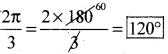Question 4.
Evaluate 7! – 5!
7! – 5! = (7 × 6 × 5 × 4 × 3 × 2 × 1) – (5 × 4 × 3 × 2 × 1) = 5040 – 120 = 4920Question 5.
Find the 20th term of the GP. $$\frac{5}{2}, \frac{5}{4}, \frac{5}{8}, \ldots \ldots$$Question 6.
Find n if nC7 = nC6
If nC7 = nC6
∴ n = 7 + 6 = 13 (∵ nCr = nCr-1).Question 7.
Find the slope of the joining the points (3, 2) and (-1, 4)
Slope of the line = m = $$\frac{y_{2}-y_{1}}{x_{2}-x_{1}}=\frac{(4)-(2)}{(-1)-(3)}=\frac{2}{-4}=\left[\frac{-1}{2}\right]$$

Question 8.
Evalaute $$\lim _{x \rightarrow 0} \frac{\cos x}{x-x}$$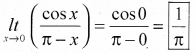Question 9.
Write the negation of the statement “√2 is a complex number. ”
“√2 is not a complex no.”

Question 10.
If $$\frac { 2 }{ 11 }$$ is the probability of an event, then what is the probability of the event ‘not
P(not A) = p(Ā) = 1 – $$\frac { 2 }{ 11 }$$ = $$\left[\frac{9}{11}\right]$$

Part – B

Answer any TEN Questions. (10 × 2 = 20)

Question 11.
If A and B are two disjoint sets and n(A) = 15 and n(B) = 10. Find n(A ∪ B) and n(A ∩ B).
n(A ∩ B) = 0
∵ A and B are disjoint net
∴ n(A ∪ B) = n(A) + n(B) = 15 + 10 = 25.

Question 12.
If U = {x : X ≤ 10 and x ∈ N} is the universal set and A = {x : X ∈ N and x is prime}, B = {x : X ∈ N and x is even} are the subsets of U, find A ∩ B’ in Roster form.
u = {1,2,3,4,5,6,7,8,9,10}, A = {2,3,5,7), B = {2,4,6,8,10,….}
∴ B’ = {1,3,5,7,9} ⇒ ∴ A ∩ B’ ={3,5,7}.

Question 13.
If A = {1,2} B = (3,4), write A × B How many subsets will A × B have?
A × B = {(1,3), (1,4),(2,3),(2,4)} and the number of subset = 2m×n = 22×2 = 16Question 14.
Find the value of sin 75°.
sin 75° = sin (45° +30°) = sin Acos B + cos Asin B = sin 45o cos 30° + cos 45osin 30°Question 15.
Find the radius of the circle in which a central angle of $$\frac{\pi}{3}$$ radians intercepts an arc of length 37.4 cm (use π = $$\frac{22}{7}$$)Question 16.
Express $$\frac{1+3 i}{1-2 i}$$ in the form of a + ib.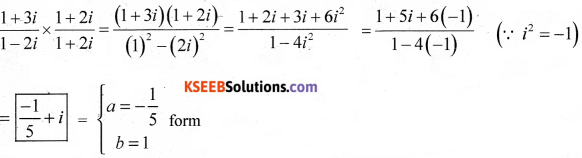Question 17.
Solve 7x + 3 < 5x + 9. Show the graph of the solution on number line.
7x + 3 < 5x + 9. ⇒ ∴ 7x – 5x < 9 – 3 ⇒ 2x < 6 ⇒ ∴[x < 3] x ∈ (-∞,3).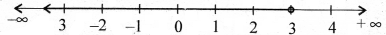Question 18.
Find the equation of the line parallel to the line 3x – 4y + 2=0 and passing through the point (–2, 3)
Line parallel to 3x – 4y + 2 = 0 can be assumed on
3x – 4y + k = 0 ∴ at (-2,3)
3(-2) – 4(3) + k = 0
∴ -6 -12 + k = 0
∴ k =18
∴ the line parallel to a given line is
3x – 4y + 18 = 0.Question 19.
Find the distance between the parallel line 15x + 8y – 34= 0 and 15x + 8y + 31 = 0
Distances between the parallel line is given byQuestion 20.
Find the distance between the points (-3,7,2) and (2,4,-1)
d = $$\sqrt{(-3-2)^{2}+(7-4)^{2}+(2+1)^{2}}=\sqrt{25+9+9}=\sqrt{43}$$

Question 21.
Evaluate $$\lim _{x \rightarrow 2}\left(\frac{x^{3}-2 x^{2}}{x^{2}-5 x+6}\right)$$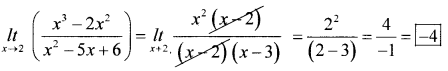Question 22.
Write the converse and contrapositive of the statement “If the two lines are parallel then they do not intersect in the same plane.
P : the two lines are paraallel V : line do not internet in the some plane.
∴ converse = q → p = If the two lines do not internet in the same plane then they are parallel.
Contrapositive: If the two lines intersect in the same plane they are parallel.

Question 23.
If the coefficient of variation and standard deviation of the distributions are 60 and 21 respectively, find the arithmetic mean of the distribution.
C.V. = 60, σ = 21, X̄ = 2, Formula: C.V.= $$\frac{\sigma}{\bar{X}} \times 100$$
60 = $$\frac{21}{\bar{X}} \times 100$$ ⇒ ∴ X̄ = $$\frac{21 \times 100}{60}$$ = 35

Question 24.
Three coins are tossed at once find the probability of getting atleast two heads.
3 coins tossed the sample space.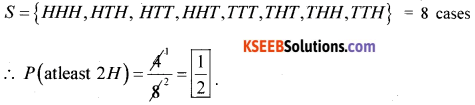Part – C

Answer any TEN of the following questions. Each question carries THREE marks. (10 × 3 = 30)

Question 25.
If U = (1,2,3,4,5,6), A = {2,3}, B = {3,4,5} show that (A ∪ B)’ = A’ ∩ B’
LHS = (A ∪ B) = {2,3,4,5}’ = {1,6}
RHS = A’ ∩ B’ = {1,4,5,6} ∩ {1,2,6} = {1,6} ∴ LHS = RHS.

Question 26.
Let f : R → R and g : R → R be defined by f(x) = x + 1, g(x) = 2x – 3, find (f + g)(x),(f – g)(x) and $$\left(\frac{f}{g}\right)$$(x)
(i) (f + g)(x) = f(x) + g(x) = (x + 1) + (2x – 3) = 13x – 21
(ii) (f – g)(x) = f(x) – g(x) = (x + 1) – (2x – 3) = x + 1 – 2x + 3 = 4 – x
(iii) (f/g)(x) = $$\left[\frac{x+1}{2 x-3}\right]$$

Question 27.
Find the general solution of the equation sin 2x + cos x = 0
Given sin 2x + cos x = 0, sin 2x = -cosx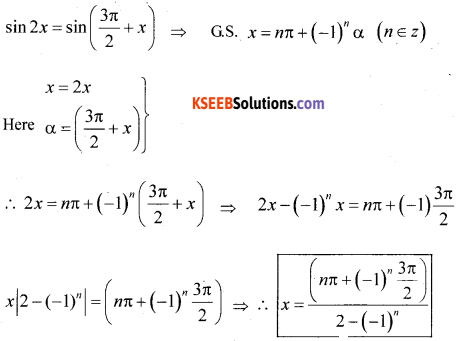sin 2x + cos x = 0, ∴ cos x=-sin 2x
cosx = cos $$\left(\frac{\pi}{2}+2 x\right)$$
∴ GS
x = 2nπ ± α (n ∈ z)
Put x = 2x ⇒ α = $$\frac{\pi}{2}$$ + 2x ⇒ x = 2nπ ± ($$\frac{\pi}{2}$$ + 2x)
(x + 2x) = (2nπ ± $$\frac{\pi}{2}$$) ⇒ 3x = 2nπ ±$$\frac{\pi}{2}$$
∴ [x = 2nπ ± $$\frac{\pi}{6}$$]

Question 28.
Express √3 + i in polar form.
(√3 + i) = [cose + isin) ….. (1)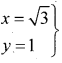r = $$\sqrt{x^{2}+y^{2}}=\sqrt{3+1}=2$$ ⇒ θ = tan-1 $$\frac{y}{x}$$ = tan-1 $$\frac{y}{x}$$ = tan-1 $$\left(\frac{1}{\sqrt{3}}\right)=\frac{\pi}{6}$$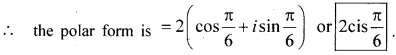Question 29.
Solve the equation x2 + 3x + 9 = 0.
GivenQuestion 30.
In how many ways can the letters of the word Permutations be arranged if (i) the words starts with P and end with S (ii) Vowels are all together. Answer:
n = 12
T repeats = 2 times ,
(i) the word starts with P and ends with S
(P ……… (5)
Total words = $$\frac{9 !}{2 !}$$ = 9 × 8 × 7 × 6 × 5 × 4 × 3 = 1,81,440 ways

(ii) Vowels are together: vowels = E,U,A,I,O = S together
∴ they form 1 group remaining $$\frac{+7}{8}$$ letters
∴ Total words = $$\frac{8 !}{2 !}$$ = 8 × 7 × 6 × 5 × 4 × 3 = 20,160 ways.Question 31.
Find the middle term in the expansion of $$\left(\frac{x}{3}+9 y\right)^{10}$$
x = $$\frac{x}{3}$$, a = 9y, n = 10, r = 5
n = 10 (even) there is only one middle term is $$\left(\frac{n}{2}+1\right)=\left(\frac{10}{2}+1\right)$$ = 6th term
General term Tr+1 = nCr xn-r ar ⇒ Ts+1 = 10C5 $$\left(\frac{x}{3}\right)^{10-5}$$ (9y)5
T6 = 10C5$$\left(\frac{x}{3}\right)^{5}$$ g5 y5 ⇒ T6 = 10C5 . $$\frac{x^{5}}{3^{5}}$$ × 95 y5 = 10C5 (xy)5 × $$\left(\frac{3^{10}}{3^{5}}\right)$$
T6 = [10C5 (xy)5 35] is the middle term.

Question 32.
Insert Five Arithmetic means between 8 and 26 such that resulting sequence is an A.P.
a = 8, an, = 26, n = 7, d = ?
Let the 5 AM’s are A1, A2, A3, A4, and A5
∴ (8), A1, A2, A3, A4, A5 (26) ⇒ Take an, = a + (n – 1) d
26 = 8 + (7 – 1)d ⇒ 18 = 6d ∴ d = 3
∴ Ar, = a + d = 8 + 3 = 11 ⇒ A2 = a + 2 = 8 + 6 =14 ⇒ A3 = 17, A4 = 20, A5 = 23.

Question 33.
In an A.P. if mth term is “n” and nth term is “m” where m ≠ n, find pth term..
Given am = n, am = m ap = ? a = ? d = ?
nth term 2,
an = a + (n – 1)d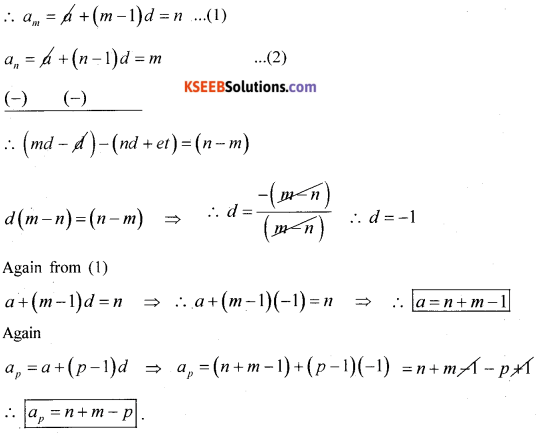Question 34.
Find the co-ordinate of the focus, equation of the directrix and length of the Latus Rectum of the parabola y2 = 8x.
Equation of parabola y2 = 8x compare with the standard form
y2 = 4ax ∴ 4a = 8 ∴ a = 2
∴ focus = (a,0) = (2,0)
Equation of directrix : x = -a.
x = -2 or [x + 2 = 0]
Latus rectum = 4a = 8.

Question 35.
Find the derivative of sin x with respect of x from first principle.
y = sin x as x → x + Δx, y + Δy
∴ (y + Δy) = sin(x + Δx)
Δy = sin(x + Δx) – y
Δy = sin(x + Δx) – sin x
÷ Δx and apply lt Δx → 0 on both side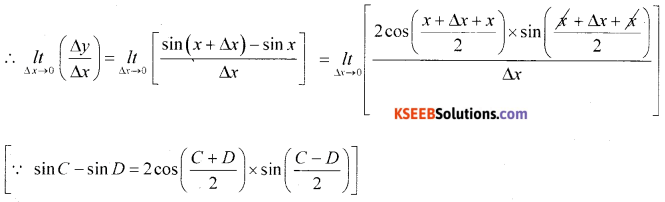Question 36.
Verify by the method of contradiction √2 is irrational
Let us assume that √2 is rational i.e.. the gives statement is false.
∴ √2 = $$\frac { 1 }{ 2 }$$ ≠ 0 a and b have no conunon factor.
Squaring ∴ 2 = $$\frac{a^{2}}{b^{2}}$$ ⇒ [a2 = 2b2] ⇒ 2 divides a
Again put a = 2C(C ∈ Z) ⇒ ∴ (2c)2 = 2b2
∴ 4c2 = 2b2 or [b2 = 2c2] 2 divide b
i.e., 2 divides both a and b hence our assumption is i.e.. a and b does flot have common contradict out statement √2 is rational is false.
∴ √2 is irrational.

Question 37.
If E and F are the evens such that P(E) = $$\frac { 1 }{ 4 }$$ , P(F) = $$\frac { 1 }{ 2 }$$ and P(E and F) = $$\frac { 1 }{ 8 }$$ . Find (i) P (E or F) (ii) P (not E and not F)
(i) P(E or F) = P(E ∪ F) = P(E) + P(F) – P(E ∩ F)
$$=\frac{1}{4}+\frac{1}{2}-\frac{1}{7}=\frac{7+14-4}{28}=\left[\frac{17}{28}\right]$$
(ii) P(E’ ∩ F’) = P(E ∪ F)’ = 1 – P(E ∪ F) = 1 – $$1-\frac{17}{28}=\frac{11}{28}$$

Question 38.
A bag contain 9 discs of which 4 are red, 3 are blue and 2 are yellow. The discs are similar in shape and size. A disc is drawn at random from the bag. Calculate the Probability that it will be (i) red (ii) yellow (iii) blue
Total ball = 4 Red + 3 blue + 2 yellow = 9 ball
(i) P (red ball) = $$\frac{4}{9}$$
(ii) P (Yellow) = $$\frac{2}{9}$$Part – D

Answer any SIX questions. (6 × 5 = 30)

Question 39.
Define a modulus function. Draw the graph and write down its domain and range.
Definition of modulus function: The function f: R → R defined by f(x) = |x|. For each x ∈ R is called modulus function. For each non-negative value of n. f(x) = x. But for negative value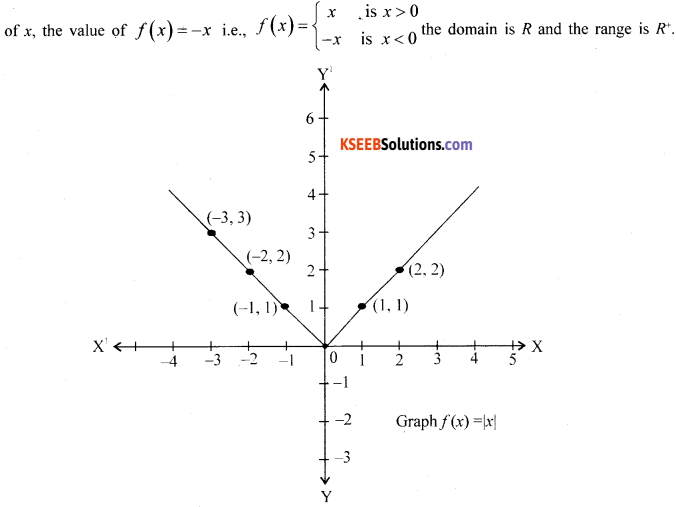Question 40.
Prove that cos2x + cos2 $$\left(x-\frac{\pi}{3}\right)=\frac{3}{2}$$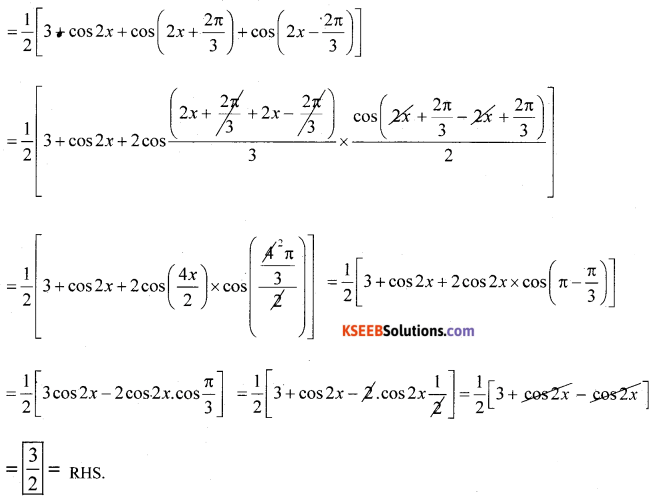Question 41.
Prove by Mathematical induction 13 + 23 + 33 + ………. + n3 $$\frac{n^{2}(n+1)^{2}}{4}$$, n ∈ N
at n = 1, LHS = RHS, $$\mathrm{l}^{3}=\frac{\mathrm{l}^{2}(1+1)^{2}}{4}$$ [1 = 1]
at n = k(+)
13 + 23 + 33 + ………. + k3 $$\frac{k^{2}(k+1)^{2}}{4}$$ ….. (1)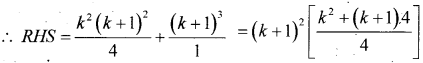Hence the given series is true for n = 1, 2, …….., k, k + 1,….. for all positive integer of n.

Question 42.
Solve the following system of inequalities graphically 2x + y ≥ 4, x + y ≤ 3, 2x – 3y ≤ 6.Question 43.
A group consists of 4 girls and 7 boys. In how many ways can a team of 5 members be selected if the team has (1) no girl (ii) atleast one boy and one girl.
Given 4 girls and 7 boy’s
(i) no girls:(ii) atleast 1 boy and 1 girls
Girls × boys
(a) 4C1 × 7C4 = 7 (b) 4C2 × 7C3 = 84.
(c) 4C3 × 7C2 = 210 (d) 4C4 × 7C1 = 140
Total number of selection 441 ways .

Question 44.
State and prove Binomial theorem for all positive integers.
Statement: (a + b)n =nC0an + nc1an-1 b + nC2 an-2 b2 + nC3 an-3 b3 + …….. + nCn-1 a.bn-1 + nCnbn
Proof: By aplying principle of mathemaical induction
Let P(n) : (a + b)n = nC0an + nc1an-1b1 + ……. + nC nbn
For n = 1 p(1) = (a + b)1 = 1C0a1 + 1C1a1-1b1
[(a + b) = (a – b)] is true
For n = K(f). p(k) = (a + b)k = kC0ak + kC1ak-1b +….+ KCkbk …..(l)
is true for n = k
We shall prove that p(k + 1) is also true i.e..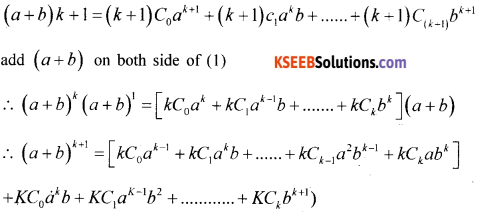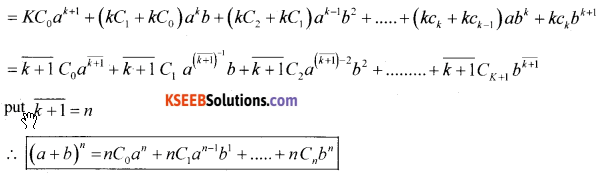Thus it has been proved that R(k + 1) is tense for p(K) is true and it is that for all positive integer n.Question 45.
Derive an expression for the co-ordinate of a point that divides the line joining the points A(x1, y1, z1) and B(x2, y2, z2)internally in the m : n. Hence find the co-ordinate of the midpoint of AB where A = (1,2,3) and B = (5,6,7)
Proof: Let p(x1, y1, z1) and Q(x2, y2, z2) be the given points
Let R(x, y, z) divide PQ internally in the ratio m : n
Draw PL, QM, RN perpendicular to xy-plane.
∴ PL ∥ RN ∥ QM
∴ PL, RN, QM lie in one plane
So that the points L, N, M lie in a straight line which is the intersection of the plane and XY plane.
Through the point R draw a line AB It to the line LM. The line AB intersect the line LP externally at A and the line MQ at B.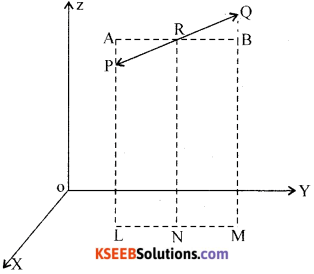Triangle APR and LIQR are similar.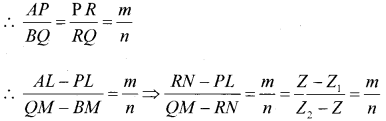∴ n(z – z1) = m(z2 – z)
∴ nz = nz1 = mz2 – m2
∴ (nz + mz) = (mz2 + nz1)
∴ z(m + n) = mz2 + nz1Question 46.
Prove that $$\lim _{\theta \rightarrow 0} \frac{\sin \theta}{\theta}=1$$ (being in radians) and hence show that $$\lim _{\theta \rightarrow 0} \frac{\tan \theta}{\theta}=1$$
$$\lim _{\theta \rightarrow 0} \frac{\sin \theta}{\theta}=1$$
Proof: Consider a circle with centre ‘O’ and radius ‘r’. Mark two point A and B on the circumference of the circle so that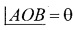At ‘A’ draw a tangent to the circle produce OB to cut the tangent at C. Joint AB.
Draw BM ⊥ OA,
Here OA = OB = r
From the figureArea of triangle OAB < area of the sector AOB < area of triangle OAC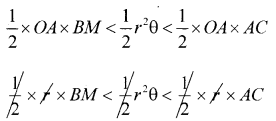∴ BM < rθ < AC … (1)
In triangle OBM : sinθ = $$\frac{B M}{O B}$$
∴ BM = OB sinθ = rsinθ
In triangle OAC: tan θ = $$\frac{AC}{O A}$$ ∴ AC = OA tan θ = r tan θ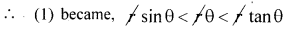sin θ < 0< tan θ
÷ sin θ
$$1<\frac{\theta}{\sin \theta}<\frac{1}{\cos \theta}$$
apply lt θ → 0Question 47.
Derive the expression for the length of the perpendicular drawn from the point (x1 y1) of the line ax + by + c = 0.
Given ax + by + c = 0
∴ ax + by = -c
÷ C .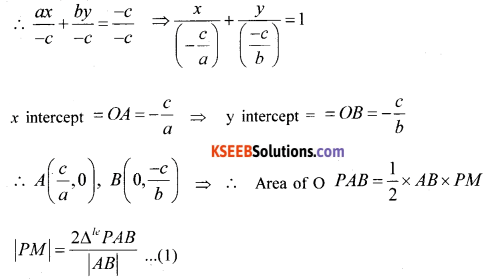P(x, y)

Question 48.
Find the mean deviation about mean for the following data: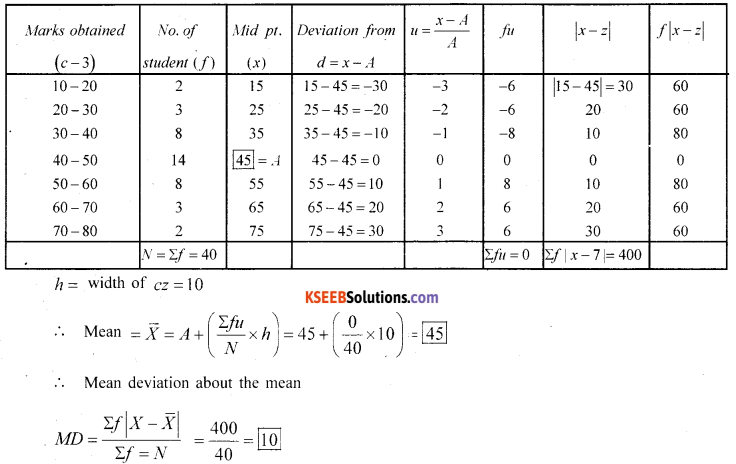Part – E

Answer any ONE question. (1 × 10 = 10)

Question 49.
(a) Prove Geometrically that cos(x + y) = cos x cos y.sin x sin y
Prove that cos(x + y) = cos x cos y.sin x sin yProof Consider a unit circle (radius = 1 unit) with centre is (0, 0).
Consider 4 point P1, P2, P3 and P4The co-ordinate of P1, P2, P3 and P4 are given by
P1 = (cosx,sinx) P2 = [cos(x + y). sìn(x + y)]
P3 = [cos(-y) sin(-y)] P4 = [1,0]
From the figure OP1OP3 is congruent to ∆P2OP4
∴ From distance formula
P1p3 = P2P4 …… (1)
Takè the distance (P1P3)2 = [cosx – cos(-y)]2 + [sin x – sin (-y)]2
= (cosx – cosy)2 + (sin x + sin y)2
= cos2x + cos2y = cosxcosy + sin2x + sin2y + 2sin cosy = 1 + 1 + 2(cosxcosy – sinxsiny)
(p1, P3)2 = 2 – 2 cos (x + y)
Again (P2P4)2 = [1(a-b)2-cos(x+y)]2 + |q – sin (x + y)|2
= 1 + cos2(x + y) -2cos(x + y) + sin2(x + y) = 1 + 1 – 2cos(x + y) = 2 – 2cos(x + y)⇒ LHS = RHS
∴ [cos(x + y) = cosx cosy – sin x sin y]

(ii) Show that cos 2x = cos2x – sin x2x
Take cos(x + y) = cos xcos y – sin xsin y
Put y = x
∴ cos(x + x) = cosx cosx – sinx sinx
cos2x = cos2 x – sin2x

(b) Find the sum of the series 5, 55, 555, 5555, … to n terms.
Sn = 5 + 55 + 555 + ……… to n term
Sn = 5[1 + 11 + 111 + ………to n term]
$$\frac{S_{n}}{5}$$ = 1 + 11 + 111 +……. to n term.
Multiply both side by 9
∴ $$\frac{9 S_{n}}{5}$$ = 9 + 99 + 999+ ………. n term
$$\frac{9 S_{n}}{5}$$ = (90 – 1) + (100 – 1) + (1000 – 1) +
$$\frac{9 S_{n}}{5}$$ = (10 + 100 + 1000 + … nth) GP (-1 – 1 – 1 … + n term)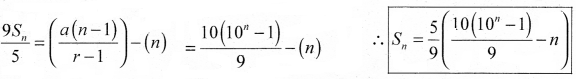Question 50.
(a) Define ellipse as a set of all points in the plane and derive its equation as $$\frac{x^{2}}{a^{2}}+\frac{y^{2}}{b^{2}}=1$$
Let F2 and F2, be the focii. ‘O’ be the mid point of the line segment F1F2. ‘0 be the origin. And a line from O through F2, be + ve and F1 be -ve ∴ the co-ordinate of F1(C1 .0) and F2 (c2 o)Let p(x,y) be a locus on the ellipse.
∴ PF1 + PF2 = 2a
Using distance formulaSquaring on both side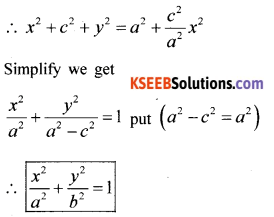(b) Find the derivative of $$\frac{x^{3}-\cos x}{\sin x}$$ with respect to X.